EXPERIMENT NO. :8

Study of End fire array antenna

Practical Session Plan

 Time ( min) Content Learning Aid / Methodology Faculty Approach Typical Student Activity Skill / Competency Developed 10 Relevance and significance of experiment Chalk & Talk , Presentation Introduces, Facilitates, Monitors Listens, Participates, Discusses Knowledge, Communication, intrapersonal 20 Explanation of experiment Chalk & Talk , Presentation Introduces, Facilitates, Explains Listens Knowledge, Communication, intrapersonal, Application 10 Calculations N/A Explains, Monitors Participates, Discusses Knowledge, Communication, Intrapersonal, Application 50 Programming Demonstration Explains, Monitors Participates, Discusses Debate, comprehension, Hands on experiment 10 Debugging & Simulating Demonstration Explains, Monitors Participates, Discusses Hands on experiment 10 Results and conclusions Keywords Lists, Facilitates Listens, Participates, Discusses Knowledge, Communication, intrapersonal, Comprehension 10 Oral Question Discussion Discussion Discussion Identifies & Participates Knowledge Intrapersonal

Title: Study of End fire array antenna.

Objectives: Model the End fire Array to analyze the performance parameters

Aim:

Â· To sketch the radiation pattern of End Fire Array with uniform spacing & amplitude

Â· To compute performance parameters

Apparatus: Matlab software, PC, Printer.

Theory:

The end fire array is nothing but broadside array except that individual elements are fed in, out of phase. Thus in the end fire array, a number of identical antennas are spaced equally along a line and individual elements are fed with currents of equal magnitude but their phases varies progressively along the line in such a way as to make the entire arrangement substantially unidirectional. In other words, individual elements are exited in such a manner that a progressive phase difference b/w adjacent elements becomes equal to the spacing b/w the elements.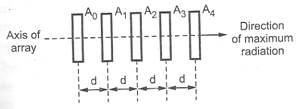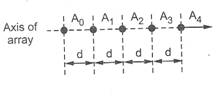Fig. 1 a Top View Fig.1b Front View

End Fire Array

End fire array defined as: the arrangement in which the principal direction of radiation coincides with the direction of the array axis.

It may be noted that end fire array may be bidirectional also.

Array of n elements with equal spacing and currents equal in Magnitude but with progressive phase shift:

Consider n no. Of identical radiators supplied with equal current which are not in phase, as shown in figure. Assume that there is a progressive phase lag of Î²d radians in each radiator.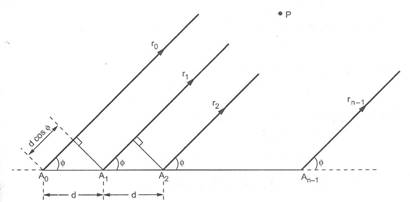Fig. 1 End Fire Array

Consider that the current supplied to first element A0 be I 0. Then the current supplied to A1 is given by,

I1= I0*e-jÎ²d

Similarly the current supplied to A2 is given by,

I2 = I1*I0*e-jÎ²d = [I 0*e-jÎ²d] e-jÎ²d= I0*e-2jÎ²d

Thus the current suppliedto last element is given by,

In-1 = I0*e-j (n-1)Î²d

The electric field produced at point P due to A0 is given by....... (1)

The electric field produced at point P due to A1 is given byBut r1 = r0 â€“ d cosÎ¦E1 = E0*e jÎ²d(cosÎ¦-1) .........(2)

Let Î¨= Î²d(cosÎ¦-1)E1 = E0 e jÎ¨ ......... (3)

The electric field produced at point P due to A2 is given by

E2 = E0 e j2Î¨ ......... (4)

Similarly, the electric field produced at point P due to An-1 is given by,

En-1 = E0 e j(n-1)Î¨ ......... (5)

Thus the resultant field at point p is given by,

ET = E0+E1+E2+.......+E n-1ET = E0+E0 e jÎ¨+ E0 e j2Î¨ ..........+ E0 e j(n-1)Î¨ET = E0 [1+ e jÎ¨+ e j2Î¨ ..........+ e j (n-1) Î¨]

Using the concept of exponential series, we can writeConsider only magnitude, we get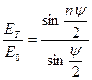In end fire Array the maximum radiation can be obtained along the axis of uniform end fire array if the progressive phase shift Î± between the elements is given by,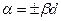= -Î²d For maximum in Î¦ = 0Â° direction

= +Î²d For maximum in Î¦ =180Â° direction

ALGORITHM:

1. Read values of NUMBER OF ARRAY ELEMENT (N), FREQUENCY (F), DISTANCE

BETWEEN ELEMENTS (d)

2. Calculate WAVELENGTH Î» = C/F WITH (c=3*108m/sec).

3. Enter the distance between Elements d.

4.Calculate AF.

4. Plot polar graph Î¦ vs. AF.

5. Calculate non-normalized AFâ€™ = N*AF.

6. The calculate AF in dB scale. = AFdn

7. AFavg= (AFdn+ AFâ€™) / 2

8. Plot polar graph Î¦ vs. AFavg

9. Calculate FNBW, HPBW, FSLBW using given formulae.

Matlab Code For End Fire Array:-

close all;

clc;

%program to plot endfire side array %

lambda=0.1;

N=input('enter the number of elements :');

k=2*pi/(lambda);

d=lambda;

beta=-k*d;

theta=0:pi/360:2*pi;

psi=k.*d.*cos(theta) + beta;

AF=(1/N)*((sin(N.*psi/2))./sin((psi./2)));

AF1=abs(AF);

%plot(theta,10*l%

plot(theta *180/pi,10*log10(abs(AF)));

grid on

xlabel('theta in degree(0 to 2pi) ')

ylabel('amplitude in db')

title('endfire linear plot ')

%polar plot

figure(2);

polar(theta,abs(AF));

title('polar plot endfire 0 to 2pi')

grid on

figure(3);

U=(abs(AF)./max(abs(AF))).^2;

polar(theta,U);

title('polar plot endfire 0 to 2pi')

grid on

Results:

Expected Matlab Output:Conclusion:

In END-FIRE ARRAY ANTENNA the maximum radiation is always parallel to the axis of antenna array i.e. at either 0Âº OR 180Âº and the maximum radiation can be obtained by increasing the number of elements..

Upon completion of experiment students will be able to :

Students will able to model the End fire antenna, compute the performance parameters and compare the performance parameters with standard parameters.

Oral Question Bank

Theory Question Bank

 Q. No Description 1 What is an â€œarray factorâ€? 2 What are the types of antenna arrays? 3 Define the length of an array 4 What are the applications of arrays? 5 What is the direction of maximum radiation is maximum only in one direction that is in the direction of array axis. 6 Define antenna array. 7 What is an array and mention the various forms of antenna arrays? 8 What is array factor or space factor? 9 Write the principle of pattern multiplication? 10 Define HPBW? 11 Define FBR? 12 Define BWFN? 13 An array consisting a no. of equidistant dipoles of equal size, fed with the same currents and phase is called:(a) end fire array (b) back fire array (c) broad side array (d) binomial array 14 Define FSLBW? 15 What are the types of antenna arrays? 16 Define the length of an array? 17 What is array factor or space factor? 18 What are the applications of arrays? 19 What is a collinear array? 20 Which are the controlling parameters of the overall pattern of antenna array? 21 Why antenna arrays are required? 22 What is uniform linear array? 23 What is the effect of changing the number of element on the radiation pattern of an antenna array? 24 What is the effect of changing the spacing between adjacent elements on radiation pattern of an antenna array?